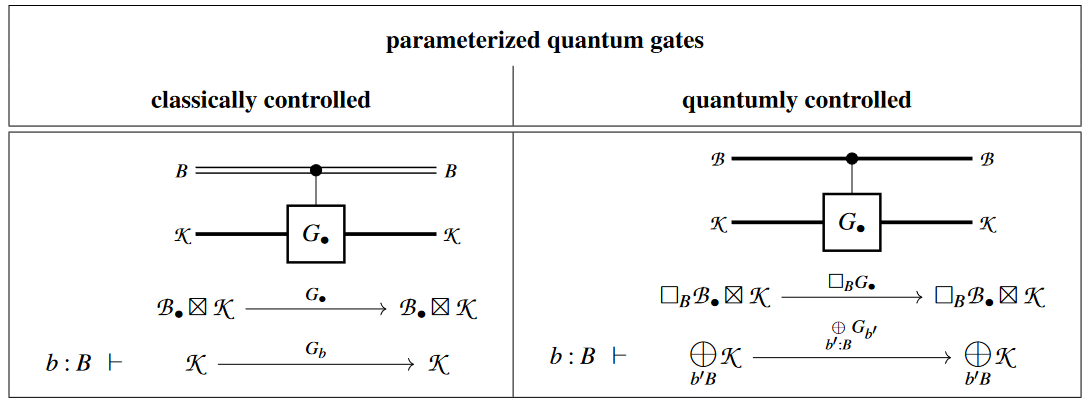# nLab controlled quantum gate

Contents

### Context

#### Computation

intuitionistic mathematics

# Contents

## Idea

In quantum information theory, a controlled quantum gate is a quantum gate whose operation on a given space of states is conditioned on the data in another space of “control states”.

### The conceptual problem

The analogous situation for classical logic gates is without subtlety: Here the parameterization of a logic gate on $(n_{in}, n_{out})$-bits by $n_{ctrl}$ control bits is a choice of function

$Bool^{n_{ctrl}} \xrightarrow{\;\;\; F_{\bullet} \;\;\;} Map \big( Bool^{n_{in}} ,\, Bool^{n_{out}} \big)$

from the intended set of controls to the set of functions between the intended input/output; and by the internal hom-adjunction in the cartesian monoidal category of Sets this is in bijective correspondence with a single function out of the Cartesian product set of the control bits with the input bits:

$\array{ Bool^{ n_{ctrl} + n_{in} } &\simeq& Bool^{ n_{ctrl} } \times Bool^{ n_{in} } &\xrightarrow{\;\;\;}& Bool^{ n_{out} } \\ && ( b_{ctrl}, b_in ) &\mapsto& F_{b_{ctrl}}\big( b_{in} \big) } \,.$

This function would/could be called the “controlled logic gate”. Notice that the control bits in the above function are “discarded after use”, in that they cannot in general be recovered from the output of the above function.

The subtleties with generalizing this situation to quantum logic gates are:

1. The control of quantum gates may be either by quantum data or by classical data, which is not quite the same.

(In a quantum-controlled quantum logic gate, the control itself may be in a quantum superposition, while a classically-controlled quantum gate is effectively an indexed set of quantum gates, indexed by classical data.)

2. A quantum-controlled quantum gate should, like any pure quantum logic gate, be unitary, in particular invertible, hence it “must not discard” its control qbits.

This rules out the otherwise evident non-cartesian analog of the above classical situation (with the product operation replaced by the non-cartesian tensor product of finite-dimensional Hilbert spaces, typically, and hence with the internal hom now being the corresponding linear space of linear maps)):

$\frac{ QBit^{n_{ctrl}} \xrightarrow{\;\;\; F_\bullet \;\;\;} Map \big( QBit^{ n } ,\, QBit^{ n } \big) }{ QBit^{n_{ctrl}} \otimes QBit^{n} \xrightarrow{\phantom{----}} QBit^{ n } }$

While the linear map at the bottom always exists, it cannot be invertible (unless the control is trivial, with $n_{ctrl} = 0$, or the state space is trivial, with $n = 0$) and hence does not qualify as a quantum logic gate.

A general and precise definition of controlled quantum gates is hard to find in traditional literature (cf. Gurevich & Blass (2021) §1).

What traditional texbooks state (e.g. Nielsen & Chuang (2000) §4.3) is that for

$G \;\colon\; \mathscr{K} \longrightarrow \mathscr{K}$

a given quantum logic gate, its version controlled by a single qbit is its direct sum with the identity map, which may be thought of as the block-diagonal matrix-notation with diagonal blocks being $G$ and the identity function:

$\underset{ QBit }{ \underbrace{ (\mathbb{C} \oplus \mathbb{C}) } } \otimes \mathscr{K} \;\simeq\; \mathscr{K} \oplus \mathscr{K} \xrightarrow{ \; id \oplus G \,=\, \left[ \begin{array}{cc} id & 0 \\ 0 & G \end{array} \right] \; } \mathscr{K} \oplus \mathscr{K} \;\simeq\; \underset{ QBit }{ \underbrace{ (\mathbb{C} \oplus \mathbb{C}) } } \otimes \mathscr{K}$

If we understand, as usual, that

$QBit \;\;\simeq\;\; \mathbb{C}\cdot \vert 0 \rangle \;\oplus\; \mathbb{C}\cdot \vert 1 \rangle$

then this gives a (invertible!) quantum gate on the tensor product space $QBit \otimes \mathscr{K}$, with the property that

1. $\vert 0 \rangle \,\otimes\, \vert \psi \rangle \;\;\mapsto\;\; \vert 0 \rangle \,\otimes\, \phantom{G}\vert \psi \rangle$

2. $\vert 1 \rangle \,\otimes\, \vert \psi \rangle \;\;\mapsto\;\; \vert 0 \rangle \,\otimes\, G\vert \psi \rangle$

In words: “If the control Qbit is definitely set, then we operate with $G$, if it is definitely not set then we operate trivially, and in general we operate with the respective superposition of these two actions.

A basic example of this construction is the controlled quantum NOT gate.

## Definition

The following is a more general definition along the lines of quantum circuits via dependent linear types.

Given a (finite) set $B$ of “control parameter values” (typically $B =$ Bool = $\{0, 1\}$), a $B$-indexed set of quantum logic gates is a morphism

$\begin{array}{rccc} & \mathscr{H}_\bullet & \xrightarrow{\;\;\; G_\bullet \;\;\;} & \mathscr{H}_\bullet \\ b \colon B \;\; \vdash \; & \mathscr{H}_b & \xrightarrow{\;\;\; G_b \;\;\;} & \mathscr{H}_b \end{array}$

in a category $LinType_B$ of $B$-dependent linear types (typically: complex vector bundles over $B$), which is fiber-wise a linear map $G_b$ with given desired properties (invertible, unitary, …).

As such, we may call this the classically controlled quantum gate, whose classical control parameter is $b \in B$ and whose quantum gate action for a given such parameter is $G_b$.

Typically the state spaces $\mathscr{H}_\bullet$ are independent of $b \colon B$, say equal to some $\mathscr{K},$ and only the quantum gates operating on a fixed state space $\mathscr{H}$ varies with the control parameter.

In this case, if we denote by

$\mathscr{B}_\bullet \;\coloneqq\; (p_B)^\ast \mathbb{1} \,,\;\;\; \mathscr{B} \;\coloneqq\; \Box_B \mathscr{B}_\bullet$

the quantum state space(s) of the control parameter, then a classically $B$-controlled quantum gate is equivalently a morphism in $LinType_B$ between the external tensor products of $\mathscr{B}_\bullet$ with $\mathscr{K}$:

$\array{ & \mathscr{B}_\bullet \boxtimes \mathscr{K} & \xrightarrow{\phantom{----}} & \mathscr{B}_\bullet \boxtimes \mathscr{K} \\ b \colon B \;\; \vdash & \mathscr{K} & \xrightarrow{\phantom{--}G_b\phantom{--}} & \mathscr{K} }$

On the other hand, the corresponding quantumly-controlled quantum gate is the image of this under the necessity-comonad (in terms of quantum modal logic, see here), hence under direct sum:

(1)$\Box_B G_\bullet \;\colon\; \Box_B \mathscr{H}_\bullet \;\simeq\; \underset{b \colon B}{\bigoplus} \mathscr{H}_b \xrightarrow{ \;\;\; \underset{b \colon B}{\oplus} G_b \;\;\; } \underset{b \colon B}{\bigoplus} \mathscr{H}_b \;\simeq\; \Box_B \mathscr{H}_\bullet$## Properties

Traditional textbook accounts of controlled quantum gates:

• Chris Heunen, Jamie Vicary, around Lem. 5.61 in: Categories for Quantum Theory, Oxford University Press 2019 $[$ISBN:9780198739616$]$